# Creating and Copying formula using relative references in Excel

In our following example, we want to calculate Menu Cost(see the heading in the example) multiplying every menu’s price and quantity. We shall not create a formula for every cell, we shall create the formula for cell D4 and copy this formula to the other rows. Here we are using relative references.

### Step 1

Select the cell that will contain your formula. In our example, the cell is D4 where we shall use the formula.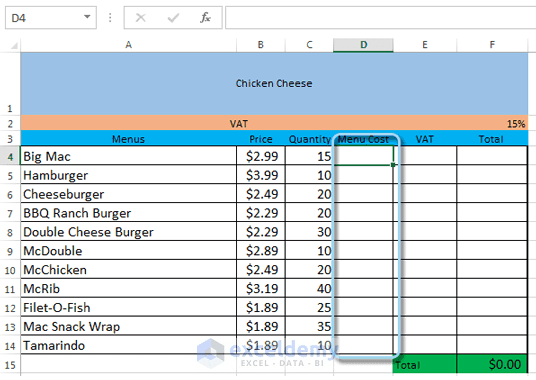Relative cell references in Excel. We shall calculate the Menu Cost using relative cell references

### Step 2

Enter your formula to calculate the desired value in the selected cell. We shall type ‘=B4*C4’ in cell D4.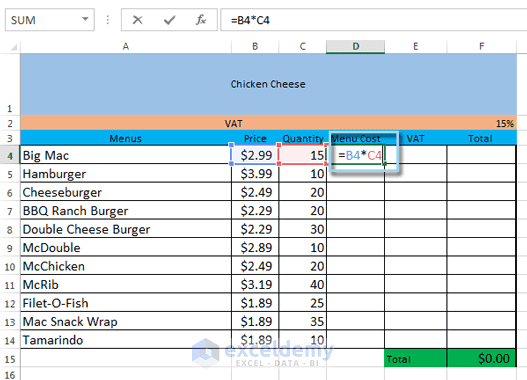Relative cell references in Excel. Entering Formula

### Step 3

Now press Enter on your keyboard. The formula will be calculated internally and you will see the calculated result in cell D4.

Read More: Relative cell references in Excel

### Step 4

By default when you pressed Enter, the active cell is now D5. Use up arrow(↑) key to make D4 as active cell again. Now locate the fill handle in the lower-right corner of the cell D4.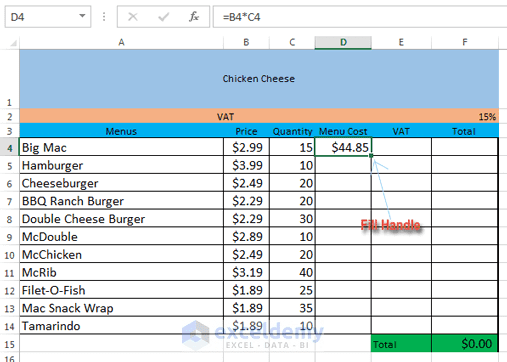Relative cell references in Excel. Fill Handle

### Step 5

Now click the fill handle, hold it and drag over the cells you wish to fill. We are selecting cells D5: D14 in our example.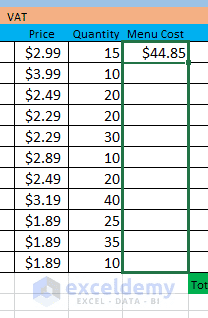Relative cell references in Excel. Fill Handle grabbing.

### Step 6

Now release the mouse. The formula, we made in cell D4, will be copied to every cell we have selected with relative references. Calculated values will be visible in every cell.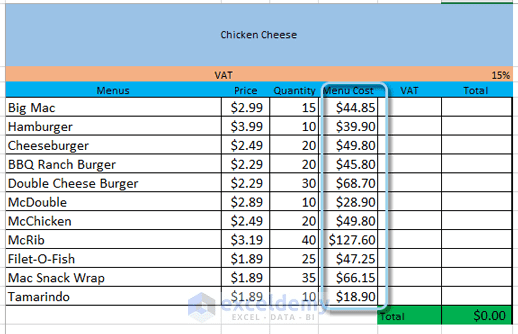Relative cell references in Excel. The formula is copied with relative cell references, values are visible now.

Note: To check whether your formula is correct, just double-click on any cell from the filled cells. You will find that this formula is similar to ‘=B4*C4’ formula, except that cell references are different and dependent on row numbers. For the row number 9, the formula is ‘=B9*C9’, just the row numbers have been changed.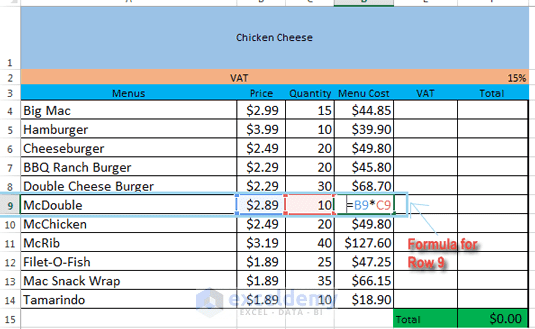Relative cell references in Excel. The formula is the same, just the cell references have been changed with the row numbers.

relative-absolute-references.xlsx

#### Kawser

Hello! Welcome to my Excel blog! It took me some time to be a fan of Excel. But now I am a die-hard fan of MS Excel. I learn new ways of doing things with Excel and share here. Not only how to guide on Excel, but you will get also topics on Finance, Statistics, Data Analysis, and BI. Stay tuned!

1 Comment